# TeaForN：让Teacher Forcing更有"远见"

## July 5, 2021 • Read: 1300 • Deep Learning • 阅读设置

#### Teacher Forcing

\begin{aligned}p(\boldsymbol{y}|\boldsymbol{x})=&\,p(y_1,y_2,\dots,y_n|\boldsymbol{x})\\ =&\,p(y_1|\boldsymbol{x})p(y_2|\boldsymbol{x},y_1)\dots p(y_n|\boldsymbol{x},y_1,\dots,y_{n-1}) \end{aligned}\tag{1}

#### 没什么远见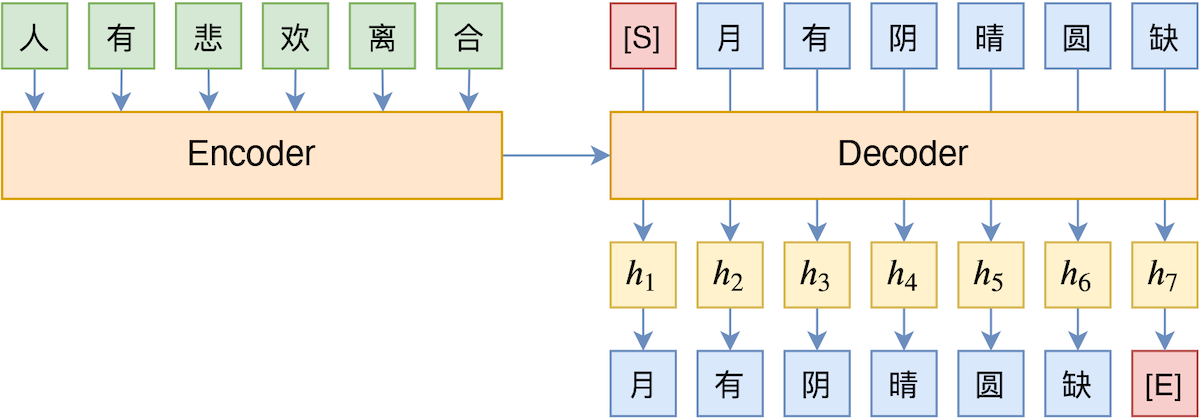Teacher Forcing示意图

1. 牺牲并行。对于Teacher Forcing来说，如果Decoder使用的是CNN或Transformer这样的结构，那么训练阶段的所有token都可以并行训练的（预测阶段还是串行），但如果Student Forcing的话则一直都是串行
2. 极难收敛。Student Forcing通常需要用Gumbel Softmax或强化学习来回传梯度，它们的训练都面临着严重的不稳定性，一般都要用Teacher Forcing预训练后才能用Student Forcing，但即便如此也不算特别稳定

#### 往前多看几步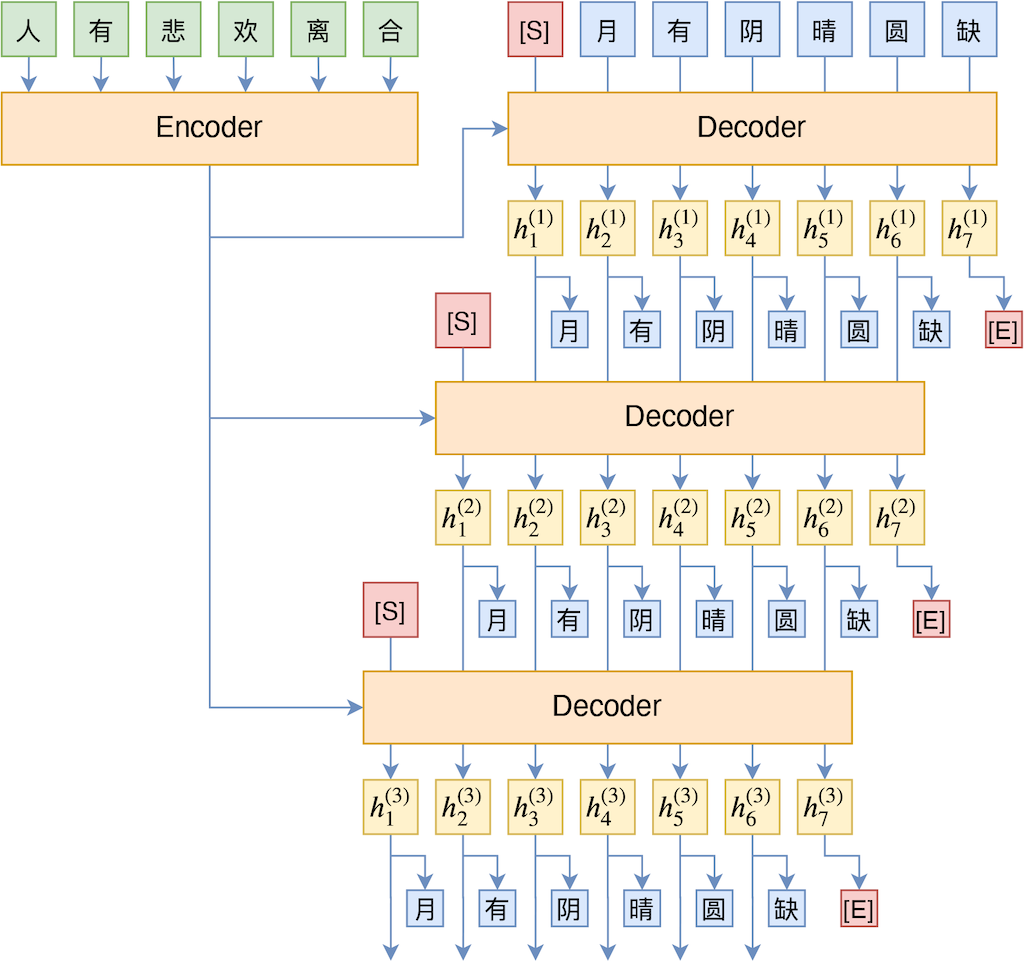TeaForN示意图

#### 用数学的话来说

$$[h_1,h_2,...,h_L]=M(E([w_0,w_1,...,w_{L-1}]))\tag{2}$$

\begin{aligned}[] \left[e_0,e_1,e_2,\cdots,e_{L-1}\right]& = E\left(\left[w_0, w_1,w_2,\cdots,w_{L-1}\right]\right)\\ \left[h_1^{(1)},h_2^{(1)},h_3^{(1)},\cdots,h_L^{(1)}\right]& = M\left(\left[e_0,e_1,e_2,\cdots,e_{L-1}\right]\right)\\ \left[h_1^{(2)},h_2^{(2)},h_3^{(2)},\cdots,h_L^{(2)}\right]& = M\left(\left[e_0, h_1^{(1)},h_2^{(1)},\cdots,h_{L-1}^{(1)}\right]\right)\\ \left[h_1^{(3)},h_2^{(3)},h_3^{(3)},\cdots,h_L^{(3)}\right]& = M\left(\left[e_0, h_1^{(2)},h_2^{(2)},\cdots,h_{L-1}^{(2)}\right]\right)\\ &\,\,\vdots \end{aligned}\tag{3}

$$\text{loss} = -\sum_{t=1}^L \sum_{i=1}^N \lambda_i \log p_t^{(i)}[w_t]\tag{4}$$

Inference阶段，我们只用$E$和$M$做常规的解码操作（比如Beam Search），也就是只用$h_t^{(1)}$而不再需要$h_t^{(2)},h_t^{(3)},...$

#### 效果、思考与讨论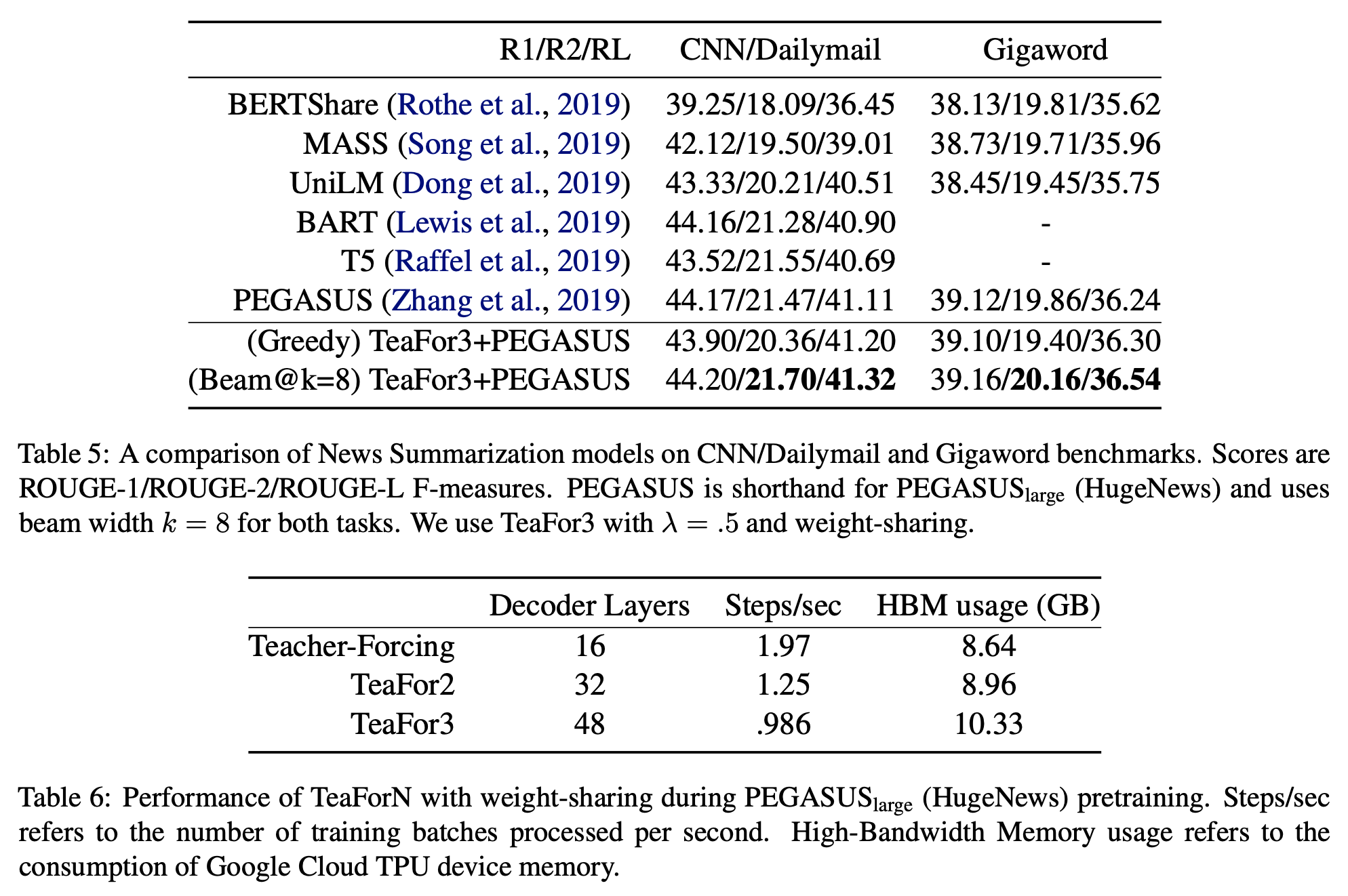TeaForN的实验结果之一（文本摘要）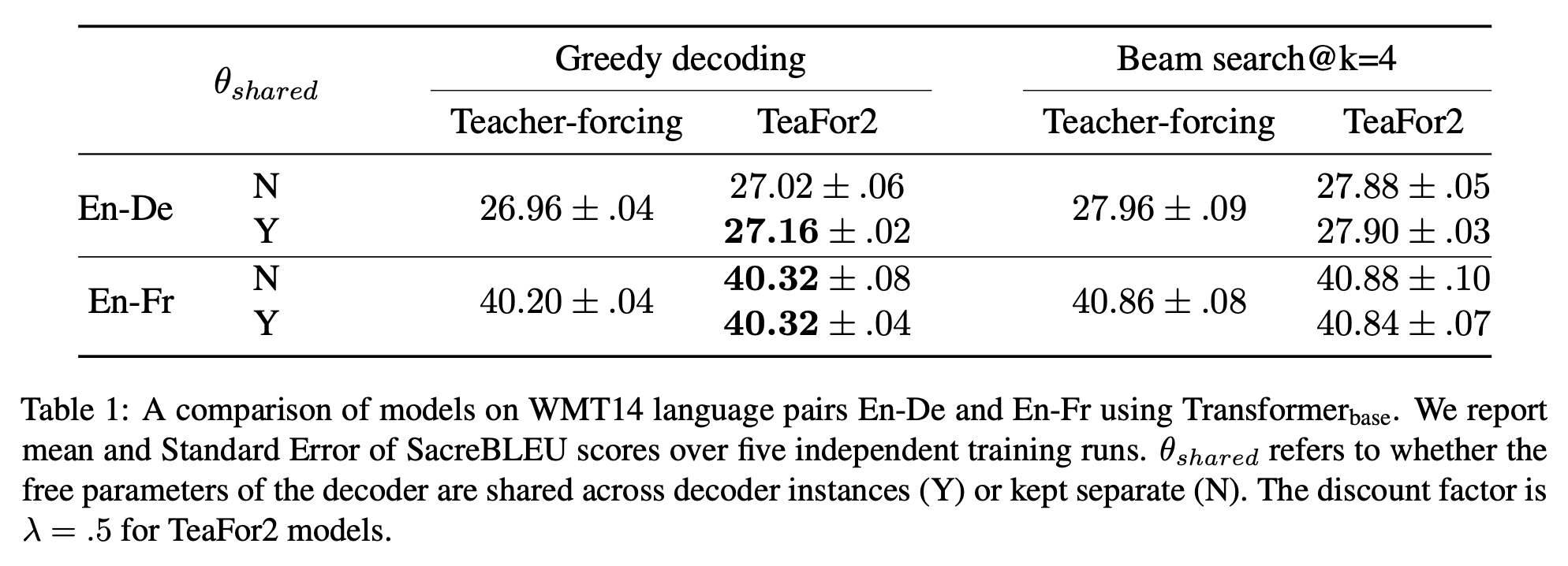TeaForN在机器翻译上的效果，其中包含了是否贡献权重的比较

$$\frac{\sum\limits_{w\in \text{Top}_k(p_t)}p_t[w] e_w}{\sum\limits_{w\in \text{Top}_k(p_t)}p_t[w]}\tag{5}$$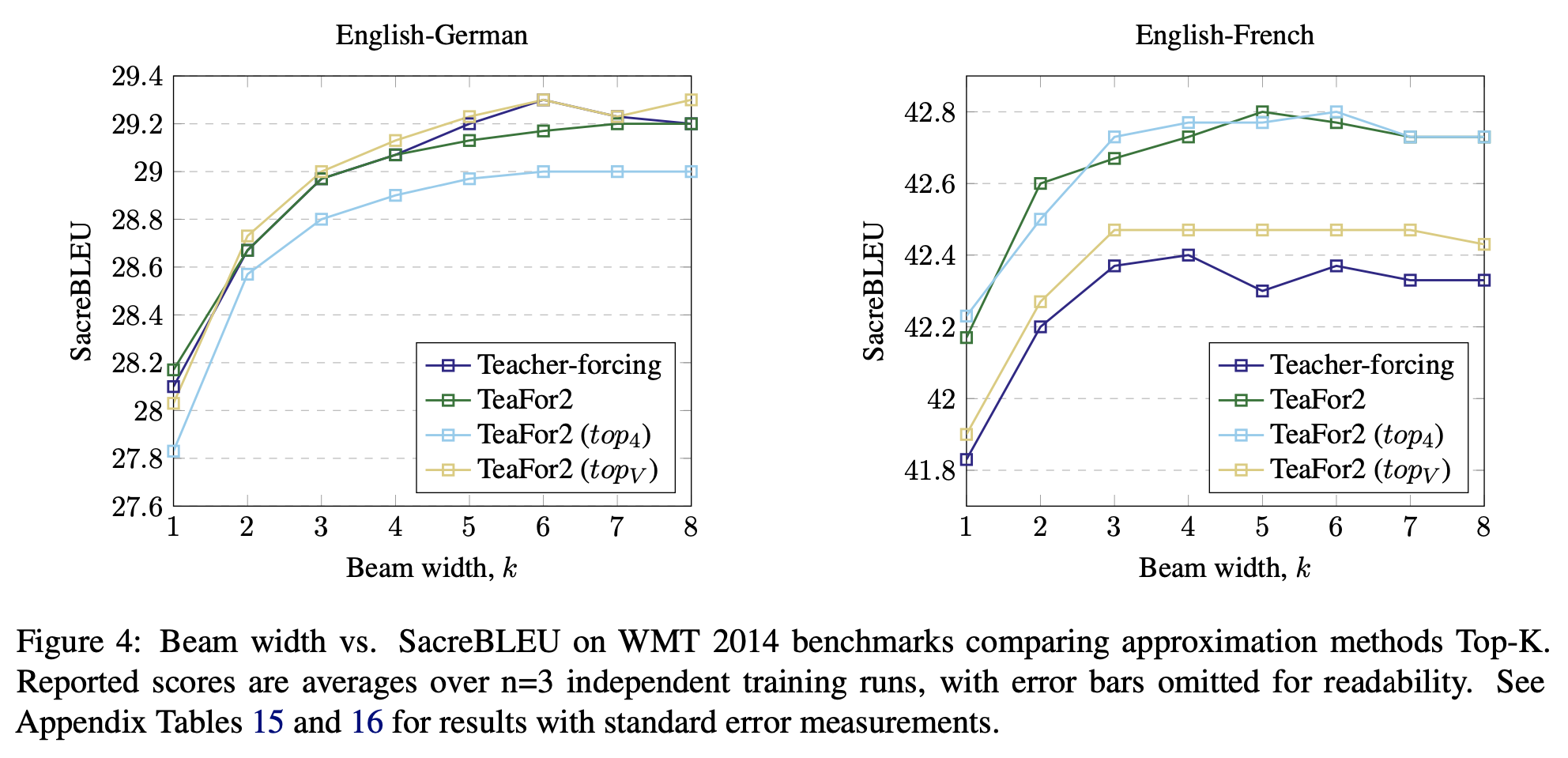#### Reference